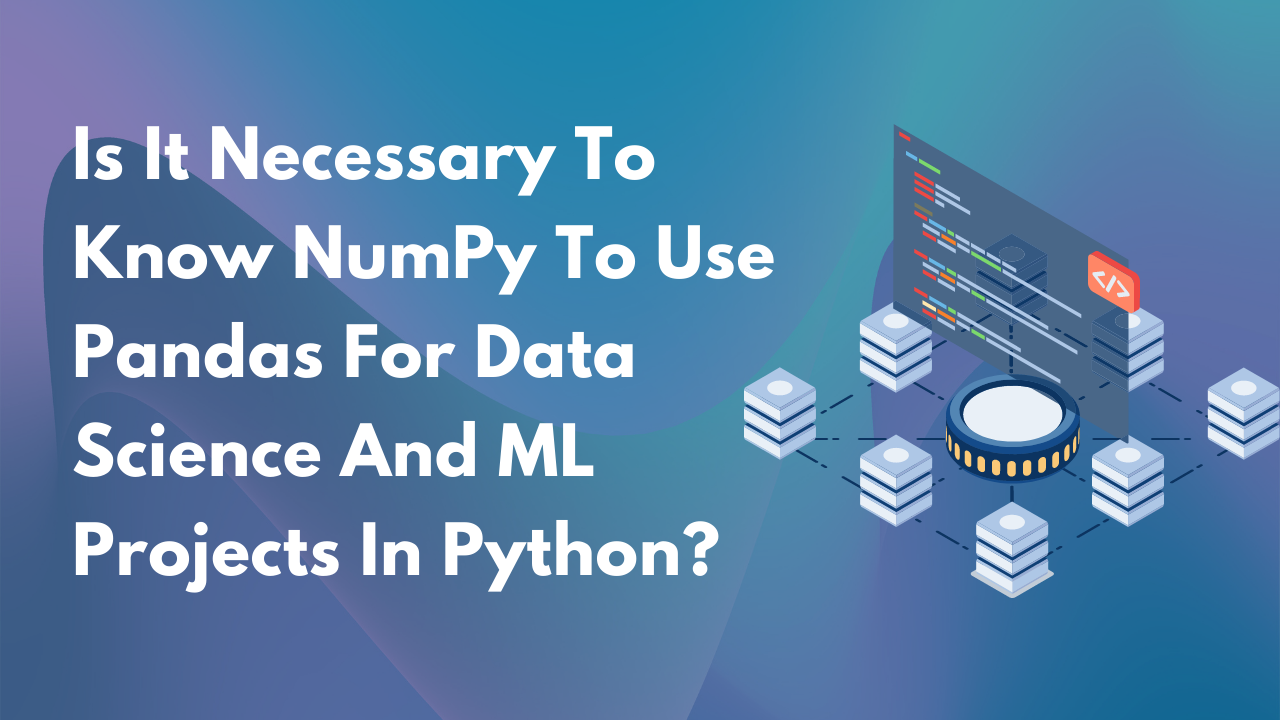# Is it necessary to know NumPy to use Pandas for data science and machine learning projects in Python?No items found.If you are new to NumPy and looking for a way to start data science with Python or machine learning, you must know that it's necessary to know about NumPy, but how far one should take it depends on what exactly you need to do. And for it, it is essential to understand first a few things about NumPy.

## What is Pandas?

Pandas is a data analysis library for the Python language. It contains various tools for processing and analyzing data in Python. NumPy stands for N-dimensional Array and is an open-source software library for scientific computing that provides an extensive collection of mathematical functions, data structures, and constants for numerical computations in Python. They are the two main packages on your path to becoming an expert Data Scientist or Machine Learning Engineer.

Pandas contain many functions that make it easy to manage your data in many ways. However, what makes Pandas particularly useful is its automatic partitioning using the core functions of NumPy, such as ndarray, numpy.transpose, numpy.reshape and numpy.where etc. So, it is essential to know about NumPy.

## What is NumPy?

NumPy, which stands for Numerical Python, is a package that works with arrays and matrices. It was initially developed by Vincent Faith and Simon Riggs as a C extension but has since become the de facto standard n-dimensional array type in modern Python environments. The library consists of low-level numerical types like array objects and high-performance libraries like ndarrays.

NumPy is often considered the best Python library for manipulating and analyzing big or dense datasets. It's a fundamental building block of many machine learning libraries, like Scikit-learn and SQLalchemy. Also, it's okay to know NumPy to use Pandas for data science or machine learning projects in Python. Several cases back the same. Even the experts at Edyst agree with it. But if you want to get outstanding results using pandas, then you should really know NumPy.

## How is NumPy used in Data Science?

NumPy is widely used in data science to perform numerical analyses and functions. One can use it to create and manipulate arrays, return descriptive statistics, and various machine learning models and mathematical formulas. NumPy generally produces array objects that are traditionally 50 times faster than the python list.

## Is Knowing NumPy to use Pandas for Data Science and Machine Learning Projects in Python Necessary?

Suppose you are still wondering why? The answer is simple. NumPy is not limited to just numeric data but can also work with other types of objects like dates or sequences of arbitrary data types with multiple elements per column. If you're working on machine learning or data mining projects in Python and need to do a lot of numerical manipulations, NumPy is your friend. Besides, a significant reason data science uses NumPy in machine learning projects in Python is to generate necessary resources quickly, which are essential. Not to mention, NumPy even uses less memory to store data. Furthermore, it even mentions the type of data stored in it.